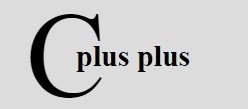# C++ Pointers and Functions

A function is a user defined operation that can be invoked by applying the call operator ( " ( ) " ) to the function's name. If the function expects to receive arguments, these arguments (actual arguments) are placed inside the call operator. The arguments are separated by commas. A function may be invoked in one of these two ways :

• call by value
• call by reference

In the first method, the 'value' of each actual argument in the calling function is copied on to the corresponding formal arguments of the called-function. The called-function works with the manipulates its own copy of arguments and because of this, changes made to the formal arguments in the called function have no effect on the values of the actual arguments in the calling function.

The second method can itself be used in two ways :

• by passing the references
• by passing the pointers

Since the call by reference method is used in two ways, we must be very much comfortable with the references and pointers.

A reference is an alias name for a variable. For instance, the following code

```float value = 1700.25 ;
float & amt = value ;      // amt reference of value```

declares amt as an alias name for the variable value. No separate memory is allocated for amt rather the variable value cna now be accessed by two names value and amt.

On the other hand, a pointer to a variable holds its memory address using which the memory area storing the data value of the variable can directly be accessed. For example, the following code

```float value = 1700.25 ;
float ∗fp = &value ;      // fp stores the address of value```

makes a float pointer ∗fp point to a float variable value. The variable value can now be accessed through fp also (using ∗fp).

The variable value can now be printed in any of the following ways :

```cout << value ;       // using variable name
cout << amt ;       // using reference name
cout << ∗fp ;        // using the pointer```

## Invoking Functions by Passing the References in C++

When parameters are passed to the function by reference, then the formal parameters become references (or aliases) to the actual parameters in the calling function. This means that (in this method) the called function does not create its own copy of original values, rather, it refers to the original values by different names i.e., their references. Thus the called function works with the original data and any change in the values gets reflected to the data.

The call by reference method is useful in situations where the values of the original variables are to be change using a function.

### Example

Let's take an example, demonstrating this concept in practical manner.

```/* C++ Pointers and Functions. This C++ program
* demonstrates about invoking the functions by
* passing the references in C++ */

#include<iostream.h>
#include<conio.h>
void swap(int &, int &);
void main()
{
clrscr();
int a, b;

cout<<"Enter any two numbers: ";
cin>>a>>b;
cout<<"\nOriginal Values:\n";
cout<<"a = "<<a<<"\tand\tb = "<<b<<"\n";
swap(a, b);
cout<<"\nValues after swapping:\n";
cout<<"a = "<<a<<"\tand\tb = "<<b<<"\n";

getch();
}
void swap(int &x, int &y)
{
int temp;
temp = x;
x = y;
y = temp;
}```

Here is the sample run of this C++ program: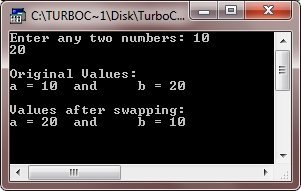## Invoking Functions by Passing the Pointers in C++

When the pointers are passed to the function, the addresses of actual arguments in the calling function are copied into formal arguments of the called function. This means that using the formal arguments (the addresses of original values) in the called function, we can make changes in the actual arguments of the calling function. Therefore, here also, the called function does not create own copy of original values, rather, it refers to the original values by the addresses (passed through pointers) it receives.

### Example

Following example will swap two values by passing addresses through pointers :

```/* C++ Pointers and Functions. This C++ program
* demonstrates about invoking the functions by
* passing the pointers in C++ */

#include<iostream.h>
#include<conio.h>
void swap(int *x, int *y);
void main()
{
clrscr();
int a, b;
cout<<"Enter two numbers: ";
cin>>a>>b;
cout<<"\nOriginal Values\n";
cout<<"a = "<<a<<" and b = "<<b<<"\n";
swap(&a, &b);
cout<<"\nSwapped Values\n";
cout<<"a = "<<a<<" and b = "<<b<<"\n";
getch();
}
void swap(int *x, int *y)
{
int temp;
temp = *x;
*x = *y;
*y = temp;
}```

When the above C++ program is compile and executed, it will produce the following output: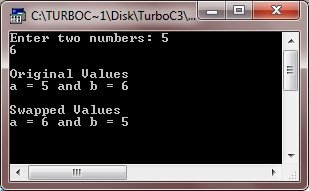The above program invokes swap() by passing addresses of a and b ; see the function call statement

`swap(&a, &b);`

&a and &b pass the addresses of a and b respectively.

The function definition receives the incoming addresses in corresponding pointers x and y.

## Function Returning by Reference in C++

A function may also return a reference i.e., an alias. Consider the following function :

```float & min(float &a, float &b)
{
if(a < b)
{
return a ;
}
else
{
return b ;
}
}```

The return type of above function min() is float & i.e., the function returns a reference to float type of variable. The above function returns reference to either a or b depending upon which of the two is lesser. It does not return any value. A function call min(x, y) will yield a reference to either a or b whichever is lesser. This means if you want to set the minimum of the two values as -1, then you may use this function on the left side of an assignment statement since it returns a reference to a variable. It will be done as shown below :

`min(x, y) = -1 ;`

The above statement assigns -1 to the lesser value of the two x and y.

Tip - A function returning a reference can appear on the left-hand side of an assignment statement.

## Function Returning Pointers in C++

The way a function can returns an int, a float, a double, or reference or any other data type, it can even return a pointer. However, the function declaration must replace it. That is, it should be explicitly mentioned in the function's prototype. The general form of prototype of a function returning a pointer would be

`type ∗ function-name(argument list) ;`

where type specifies the pointer type (int, float, double or any other data type) being returned by the function specified by function-name. The return type of a function returning a pointer must be known because the pointer arithmetic is relative to its base type and a compiler must know what type of data the pointer is pointing to in order to make it point to the next data item.

### Example

Following example program illustrates how a pointer can be returned from a function :

```/* C++ Pointers and Functions. This C++ program
* demonstrates about functions returning
* pointers in C++ */

#include<iostream.h>
#include<conio.h>
int *biger(int &, int &);
void main()
{
clrscr();
int num1, num2, *c;
cout<<"Enter two integers\n";
cin>>num1>>num2;
c = biger(num1, num2);
cout<<"The bigger value = "<<*c;
getch();
}
int *biger(int &x, int &y)
{
if(x>y)
{
return(&x);
}
else
{
return(&y);
}
}```

When the above program is compile and executed, it will produce the following output: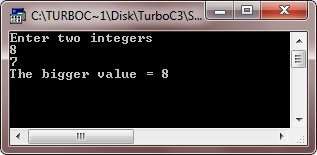The above program passes two integers by reference to the function big() that compares the two integers values passed to it and returns the memory address of bigger value. Since the return value of big() is int ∗ i.e., an int pointer, it is received in an int pointer c (Notice the statement c = big(a, b);). Then the value being pointed to by pointer c is printed on the screen.

The concept of functions returning pointers can be put to use while handling strings. Here is a program illustrating about this

```/* C++ Pointers and Functions. This C++ program
* search for a given character inside a string
* and to print the string from the point of match.
* This program demonstrates the concept of
* function returning pointers in C++ */

#include<iostream.h>
#include<conio.h>
char *match(char, char *);
void main()
{
clrscr();
char str;
char *cptr, ch;
cout<<"Enter a string\n";
cin.getline(str, 80);
cout<<"Enter a character to be searched for...";
cin>>ch;
cptr = NULL;
cptr = match(ch, str);
if(*cptr)
{
cout<<"\n";
for(; (*cptr != '\0'); cptr++)
{
cout<<*cptr;
}
}
else
{
cout<<"No match found..!!";
}
getch();
}
char *match(char c, char *s)
{
while((c != *s) && (*s))
{
s++;
}
return(s);
}```

Here is the sample run of the above C++ program: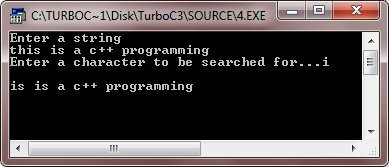Tools
Calculator

Quick Links
Signup - Login - Give Online Test